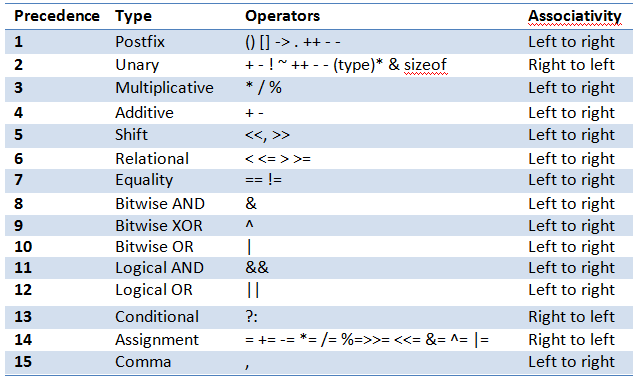# Operator Precedence and Its Associativity in C Programming

We have seen so many operators above. One can use all the operators in the same expression. but when multiple operators are used in the expressions, they cannot be evaluated from left to right or from right to left. Each one of the operators have their own priority for evaluating – precedence and the evaluation format –from left to right or right to left – called its associativity.

Below is the table shows the order of evaluation of expressions using all the operators and the associativity of their evaluation.## Let us consider an example with expression with multiple operators:

Result = (4+7*5) -30 >0 ? 1:0;

Above expression uses arithmetic operators, conditional operator, relational operator and parenthesis. In order to evaluate this expression, first we need to evaluate the expression within the parenthesis. It has arithmetic expression. Its priorities are first multiplication and then addition. Hence we need to evaluate 7*5 first and add this result with 4. Hence the result within parenthesis is 39. In order to evaluate conditional operator, we need to complete all the arithmetic operation on LHS. Hence subtract 30 from 39. It results in 9. Now check 9>0. It’s correct and returns TRUE. Hence the expression results in 1. In above expression, we have considered to evaluate the condition for conditional operator is because of its associativity is from right to left. Hence it needs to be evaluated first which in turn requires its condition to be evaluated. This condition is arithmetic which is evaluated from left to right starting from expression within parenthesis and then subtraction. This is how any expression with multiple operators are evaluated.

Translate »